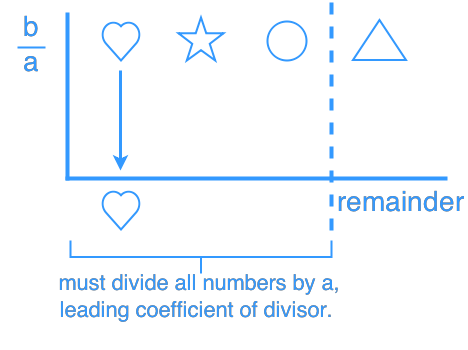# Polynomial synthetic division

### Polynomial synthetic division

Synthetic division is a shortcut method of dividing polynomials as opposed to long division. Yet, this method can only be used when we are dividing a liner expression and the leading coefficient is a 1.

#### Lessons

Synthetic division by $\left( {x - b} \right)$• 1.
Synthetic division by $\left( {x - b} \right)$
$\left( {4{x^6} - 5{x^3} + {x^2} - 9} \right) \div \left( {x - 3} \right)$
i) Operate synthetic division
ii) Write the division statement

• 2.
Synthetic division by $\left( {ax - b} \right)$
Operate synthetic division and write the division statement.
a)
$\left( {8{x^3} - 14{x^2} + 7x - 1} \right) \div \left( {2x - 5} \right)$

b)
$\left( {29{x^3} - 12{x^4} - x} \right)$ $\div$ $\left( {5 - 3x} \right)$